# 4.2 Mesh generation modules

An alphabetic list of the mesh generation modules is given below:

APNOP3:
Data
O.D.S. NOPO

Aim:
Super module  for calling some simple mesh generators and all the transformation modules for three-dimensional meshes using keywords.
Calling the module:
Via preprocessor APN3XX (see chapter 2), or in the classical manner (ditto).

APNOPO:
Data
O.D.S. NOPO

Aim:
Super module  for calling the mesh generators and transformation modules for two-dimensional meshes using keywords.
Calling the module:
Via preprocessor APNOXX (see chapter 1), or in the classical manner (ditto).

COLIB2:
Calling program or Data
O.D.S. NOPO
Aim:
2D and 3D mesh generation  by the multi-block method . The data consists of a coarse mesh of the domain consisting of a few elements on the edges of which control points are given. Each element is subsequently split by taking these points into account.

If the given mesh consists of triangles and quadrilaterals in Rł, the mesh generator creates the mesh of the corresponding surface.

Calling the module:
Via preprocessor COLIXX (when the data is relatively little), see chapter 3 or the classical method (ditto).

CONOPO:
O.D.S. NOPO
Aim:
Construct  a D.S. NOPO containing a P1 type mesh from the description of each element.

This module can be employed as an interface for non-MODULEF meshes in order to make them compatible with the modules  in this code after conversion into NOPO.

Calling the module:
The classical manner only:

Dimension array M (in the blank common), call INITI

. IAREFF,IACOOR,NFNOPS,NINOPS)

where

• M: the super array
• NDIM: the space dimension
• NE: the number of elements in the mesh
• NP: the number of points in the mesh
• IADEX: the address in M of the array describing the elements
• IAREFS: the address in M of the array containing the reference numbers of the NP vertices
• IAREFA: the address in M of the array containing the reference numbers of the edges
• IAREFF: the address in M of the array containing the reference numbers of the faces
• IACOOR: the address in M of the array containing the coordinates of the NP vertices
• NF(NI)NOPS: the support number of the O.D.S. NOPO and its level

The user must address and complete the descriptive arrays in M, their addresses being passed as arguments of the module.

The arrays to complete are the following:

• The element description with address IADEX: If NPO(i) is the is the number of vertices of element i, then the length of this array is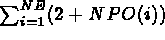. This array contains:

• Loop from i=1 to NE
• NCGE: the geometric code of the element
• NDSDE: the sub-domain number of the element
• Loop from j=1 to NPO(i)
1. the vertex numbers of element i
• end of loop j
• end of loop i

• The vertex references with address IAREFS: The length of the array is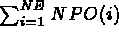. This array contains:

• Loop from i=1 to NE
• Loop from j=1 to NPO(i)
1. the reference numbers of the vertices of element i
• end of loop j
• end of loop i

• The edge references with address IAREFA: The length of the array is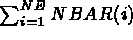where NBAR(i) is the number of edges of element i. This array contains:

• Loop from i=1 to NE
• Loop from j=1 to NBAR(i)
1. the edge reference numbers of element i
• end of loop j
• end of loop i

• The face references with address IAREFS: The length of this array is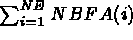where NBFA(i) is the number of faces of element i. This array contains:

• Loop from i=1 to NE
• Loop from j=1 to NBFA(i)
1. the face reference numbers of element i
• end of loop j
• end of loop i

• The vertex coordinates from address IACOOR: The length of this array is NDIM*NP. It contains the coordinates of the NP vertices:

X(1), Y(1), X(2), Y(2), ... X(NP), Y(NP)
X(1), Y(1), Z(1), X(2), Y(2), Z(2), ... X(NP), Y(NP), Z(NP)
if NDIM = 3

Remarks:

The order of the vertices, edges and faces is the standard order (see
 figure 4.1).

The module MAOBJE  is a simplified version of CONOPO with NE=1.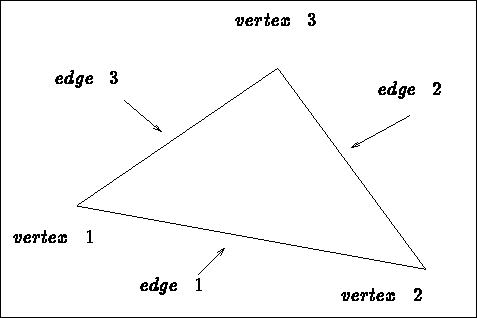Figure 4.1: Local (ordered) numbering of the items

GEL3D1:
Calling program
O.D.S. NOPO

Aim:
3D mesh generation   for hexahedral type geometries. The data consists of a distribution of points in the 3 directions corresponding to the topological cube associated with the domain.
Calling the module:
Classical call (see chapter 3).

MA2D3E:
I.D.S. NOPO (2D)
O.D.S. NOPO

Aim:
3D mesh generation  for a cylindrical type geometry. The data consists of a 2D mesh of a section representative of the domain. From the latter the different sections are defined for the construction of the layers constituting the three-dimensional mesh.
Calling the module:
Via preprocessor MA23XX ( MA2DXX in a simplified version) or in the classical manner (see chapter 3).

QUACOO:
Data
O.D.S. NOPO
Aim:
2D mesh generation  for a generalized quadrilateral type geometry by an algebraic  method. The data consists of the contour of the domain seen as 4 sides (from a topological point of view). Each side is defined as a set of characteristic lines.

The only constraint is that the number of points present on 2 opposite sides must be equal.

Calling the module:
Via keyword QUAC of APNOPO (see chapter 1).

TRICOO:
Data
O.D.S. NOPO

Aim:
2D mesh generation  for a generalized triangular type geometry by an algebraic method. The data consists of a contour of the domain seen as 3 sides (from a topological point of view). Each side is defined as a set of characteristic lines.

The only constraint is that the number of points present on the 3 sides must be equal.

Calling the module:
Via keyword TRIC of APNOPO (see chapter 1).

TN2D3D:
I.D.S. NOPO (2D)
O.D.S. NOPO
Aim:
Construct a surface mesh from a plane mesh . The vertex coordinates of the surface are calculated from the point coordinates of the starting mesh via a function called F2D3D.
Calling the module:
Via keyword SU23 of APNOP3, via preprocessor
 TN2DXX or in the classical manner (see below).

Dimension array M (in the blank common), declare the logical FONINT, call INITI

CALL TN2D3D (M,NFNOPE,NINOPE,NFNOPS,NINOPS,NTNOPS,
. FONINT,F2D3D,NTRANS)

where

• M: the super array
• NF(NI)NOPE: the support number of the I.D.S. NOPO and its level
• NF(NI)NOPS: the same for the O.D.S. NOPO
• NTNOPS: the number of arrays associated with the O.D.S. (in general 0)
• FONINT: a logical initialized to .FALSE.
• F2D3D: the formal name of the subroutine to calculate the coordinates
• NTRANS: the type of transformation: 0: mesh 2D3D ;
1: mesh 2D2D ; 2: mesh 1D2D ;

Remarks:

If NTNOPS > 0, it is necessary to input the associated arrays (see RECOLC).

Subroutine F2D3D is defined as follows:

SUBROUTINE F2D3D (X,Y,X3,Y3,Z3)

where

• X,Y: the coordinates of a point in the starting mesh
• X3,Y3,Z3: the coordinates of the 3D point derived

Modules COLIB2 and MA2D3D can also be used to construct surfaces from different data.

tn22.psfig:tn2d3dInitial meshtn23.psfig:tn2d3d1Derived surface

TRIFRO:
Data
O.D.S. NOPO
Aim:
2D mesh generation  for an arbitrary geometry by a frontal   method. The data consists of the contour of the domain composed of a set of characteristic lines.

The contour may contain several connected components. In addition, internal points can be fixed.

Calling the module:
Via keyword TRIA of APNOPO (see chapter 1).

TRIHER:
Data
O.D.S. NOPO
Aim:
2D mesh generation  for an arbitrary geometry by a Voronoi   method. The data consists of the contour of the domain seen as a set of characteristic lines.

The contour may contain several connected components. In addition, internal points can be fixed.

Calling the module:
Via keyword TRIH of APNOPO (see chapter 1).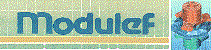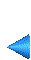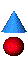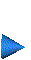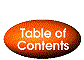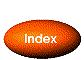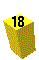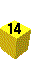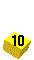Next: 4.3 Mesh modification modules Up: 4 List of the modules Prev: 4.1 Introduction Index Contents